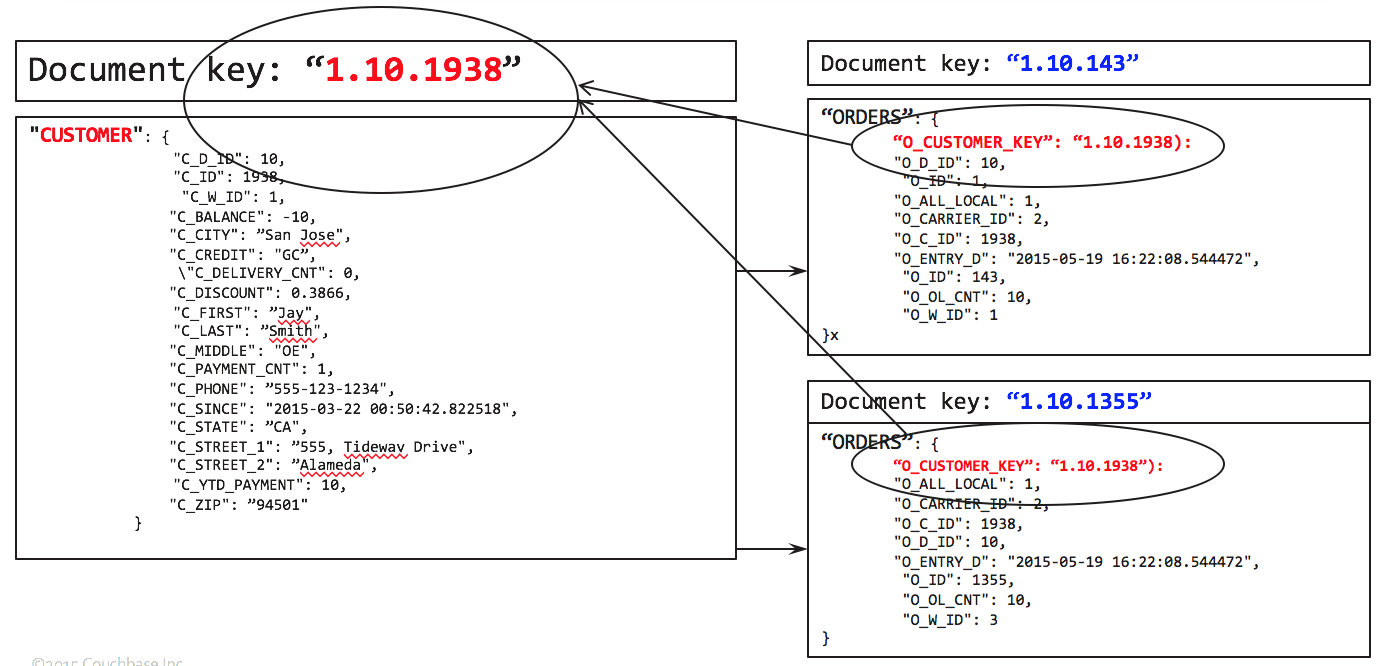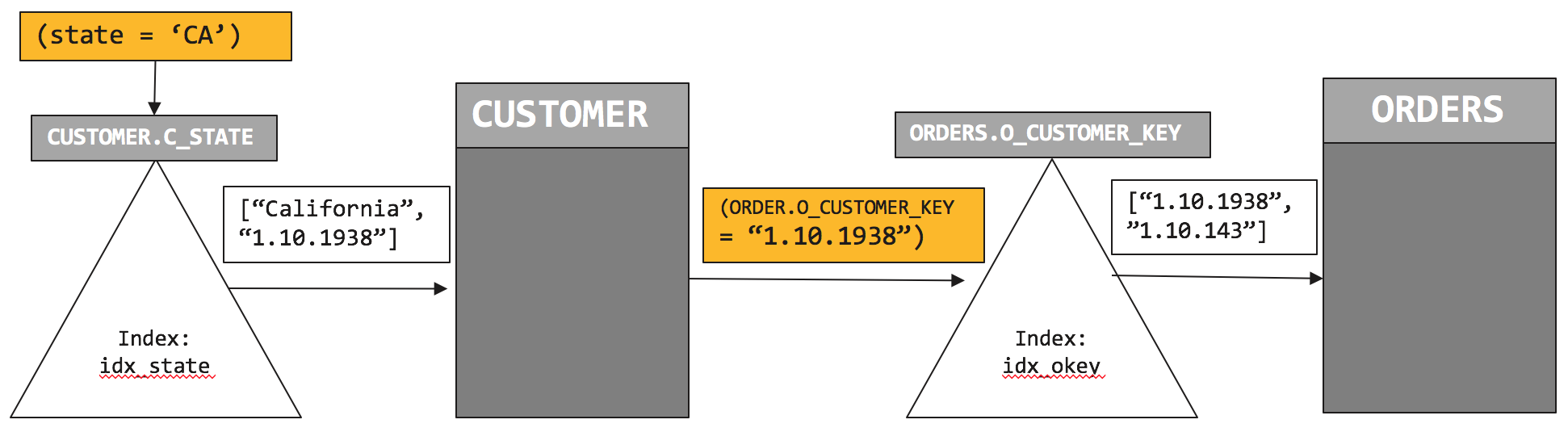## Saturday, July 30, 2016

### Join faster with Couchbase Index Joins

Good features in query language help you to optimize the data model, save space, and increase performance.
Normally, you’d have child table pointing to parent. For example, orders have the document key of the customer. So, starting with orders, you join customers to have the fully joined document which can be processed further.To get the list of orders by zipcode, you write the following query.
`SELECT c.C_ZIP, COUNT(o.O_ID)`
`FROM ORDERS AS o LEFT OUTER JOIN CUSTOMER AS c`
`         ON KEYS o.O_CUSTOMER_KEY`
`GROUP BY c.C_ZIP`
`ORDER BY COUNT(1) desc;`
This works like a charm. Let's look at the query plan.
We use the primary index on ORDERS to do the full scan.   For each document there, try to find the matching CUSTOMER document by using the ORDERS.O_CUSTOMER_KEY as the document key.  After the JOIN, grouping, aggregation and sorting follows.
`[`
`  {`
`    "plan": {`
`      "#operator": "Sequence",`
`      "~children": [`
`        {`
`          "#operator": "Sequence",`
`          "~children": [`
`            {`
`              "#operator": "PrimaryScan",`
`              "index": "#primary",`
`              "keyspace": "ORDERS",`
`              "namespace": "default",`
`              "using": "gsi"`
`            },`
`            {`
`              "#operator": "Parallel",`
`              "~child": {`
`                "#operator": "Sequence",`
`                "~children": [`
`                  {`
`                    "#operator": "Fetch",`
`                    "as": "o",`
`                    "keyspace": "ORDERS",`
`                    "namespace": "default"`
`                  },`
`                  {`
`                    "#operator": "Join",`
`                    "as": "c",`
`                    "keyspace": "CUSTOMER",`
`                    "namespace": "default",`
`                    "on_keys": "(`o`.`O_CUSTOMER_KEY`)",`
`                    "outer": true`
`                  },`
`                  {`
`                    "#operator": "InitialGroup",`
`                    "aggregates": [`
`                      "count((`o`.`O_ID`))",`
`                      "count(1)"`
`                    ],`
`                    "group_keys": [`
`                      "(`c`.`C_ZIP`)"`
`                    ]`
`                  }`
`                ]`
`              }`
`            },`
`            {`
`              "#operator": "IntermediateGroup",`
`              "aggregates": [`
`                "count((`o`.`O_ID`))",`
`                "count(1)"`
`              ],`
`              "group_keys": [`
`                "(`c`.`C_ZIP`)"`
`              ]`
`            },`
`            {`
`              "#operator": "FinalGroup",`
`              "aggregates": [`
`                "count((`o`.`O_ID`))",`
`                "count(1)"`
`              ],`
`              "group_keys": [`
`                "(`c`.`C_ZIP`)"`
`              ]`
`            },`
`            {`
`              "#operator": "Parallel",`
`              "~child": {`
`                "#operator": "Sequence",`
`                "~children": [`
`                  {`
`                    "#operator": "InitialProject",`
`                    "result_terms": [`
`                      {`
`                        "expr": "(`c`.`C_ZIP`)"`
`                      },`
`                      {`
`                        "expr": "count((`o`.`O_ID`))"`
`                      }`
`                    ]`
`                  }`
`                ]`
`              }`
`            }`
`          ]`
`        },`
`        {`
`          "#operator": "Order",`
`          "sort_terms": [`
`            {`
`              "desc": true,`
`              "expr": "count(1)"`
`            }`
`          ]`
`        },`
`        {`
`          "#operator": "FinalProject"`
`        }`
`      ]`
`    },`
`    "text": "SELECT c.C_ZIP, COUNT(o.O_ID)\nFROM ORDERS AS o LEFT OUTER JOIN CUSTOMER AS c\n         ON KEYS o.O_CUSTOMER_KEY\nGROUP BY c.C_ZIP\nORDER BY COUNT(1) desc;"`
`  }`
`]`
But, what if you’re interested California (CA) residents only? Simply add a predicate on the C_STATE field.
`SELECT c.C_ZIP, COUNT(o.O_ID)`
`FROM ORDERS AS o LEFT OUTER JOIN CUSTOMER AS c`
`         ON KEYS o.O_CUSTOMER_KEY`
`WHERE c.C_STATE = "CA"`
`GROUP BY c.C_ZIP`
`ORDER BY COUNT(1) desc;`
This works, except, we end up scanning all of the orders, whether the orders belong to California or not.  Only after the JOIN operation, we apply the C_STATE = "CA" filter.  In a large data set, this has negative performance impact.  What if we could improve the performance by limiting the amount of data accessed on ORDERS bucket.
This is exactly what the index-joins feature will help you do.  The alternate query is below.
`SELECT c.C_ZIP, COUNT(o.O_ID)`
`FROM CUSTOMER AS c LEFT OUTER JOIN ORDERS AS o`
`         ON KEY o.O_CUSTOMER_KEY FOR c`
`WHERE c.C_STATE = "CA"`
`GROUP BY c.C_ZIP`
`ORDER BY COUNT(1) desc;`
You do need an index on ORDERS.O_CUSTOMER_KEY.
To further improve the performance of this, you can create the index on CUSTOMER.C_STATE.
`CREATE INDEX idx_okey ON ORDERS(O_CUSTOMER_KEY);`
With these indexes, you get a plan like the following:
`CREATE INDEX idx_cstate ON CUSTOMER(C_STATE);`
Let's examine the explain. We use Two indexes idx_cstate which scans the CUSTOMER with the predicate (C_STATE = "CA") and then idx_okey which helps to find the matching document in ORDERS.
`[`
`  {`
`    "plan": {`
`      "#operator": "Sequence",`
`      "~children": [`
`        {`
`          "#operator": "Sequence",`
`          "~children": [`
`            {`
`              "#operator": "IndexScan",`
`              "index": "idx_cstate",`
`              "index_id": "a3a663ec9928d888",`
`              "keyspace": "CUSTOMER",`
`              "namespace": "default",`
`              "spans": [`
`                {`
`                  "Range": {`
`                    "High": [`
`                      "\"CA\""`
`                    ],`
`                    "Inclusion": 3,`
`                    "Low": [`
`                      "\"CA\""`
`                    ]`
`                  }`
`                }`
`              ],`
`              "using": "gsi"`
`            },`
`            {`
`              "#operator": "Parallel",`
`              "~child": {`
`                "#operator": "Sequence",`
`                "~children": [`
`                  {`
`                    "#operator": "Fetch",`
`                    "as": "c",`
`                    "keyspace": "CUSTOMER",`
`                    "namespace": "default"`
`                  },`
`                  {`
`                    "#operator": "IndexJoin",`
`                    "as": "o",`
`                    "for": "c",`
`                    "keyspace": "ORDERS",`
`                    "namespace": "default",`
`                    "on_key": "(`o`.`O_CUSTOMER_KEY`)",`
`                    "outer": true,`
`                    "scan": {`
`                      "index": "idx_okey",`
`                      "index_id": "271ea96d9390e10d",`
`                      "using": "gsi"`
`                    }`
`                  },`
`                  {`
`                    "#operator": "Filter",`
`                    "condition": "((`c`.`C_STATE`) = \"CA\")"`
`                  },`
`                  {`
`                    "#operator": "InitialGroup",`
`                    "aggregates": [`
`                      "count((`o`.`O_ID`))",`
`                      "count(1)"`
`                    ],`
`                    "group_keys": [`
`                      "(`c`.`C_ZIP`)"`
`                    ]`
`                  }`
`                ]`
`              }`
`            },`
`            {`
`              "#operator": "IntermediateGroup",`
`              "aggregates": [`
`                "count((`o`.`O_ID`))",`
`                "count(1)"`
`              ],`
`              "group_keys": [`
`                "(`c`.`C_ZIP`)"`
`              ]`
`            },`
`            {`
`              "#operator": "FinalGroup",`
`              "aggregates": [`
`                "count((`o`.`O_ID`))",`
`                "count(1)"`
`              ],`
`              "group_keys": [`
`                "(`c`.`C_ZIP`)"`
`              ]`
`            },`
`            {`
`              "#operator": "Parallel",`
`              "~child": {`
`                "#operator": "Sequence",`
`                "~children": [`
`                  {`
`                    "#operator": "InitialProject",`
`                    "result_terms": [`
`                      {`
`                        "expr": "(`c`.`C_ZIP`)"`
`                      },`
`                      {`
`                        "expr": "count((`o`.`O_ID`))"`
`                      }`
`                    ]`
`                  }`
`                ]`
`              }`
`            }`
`          ]`
`        },`
`        {`
`          "#operator": "Order",`
`          "sort_terms": [`
`            {`
`              "desc": true,`
`              "expr": "count(1)"`
`            }`
`          ]`
`        },`
`        {`
`          "#operator": "FinalProject"`
`        }`
`      ]`
`    },`
`    "text": "SELECT c.C_ZIP, COUNT(o.O_ID)\nFROM CUSTOMER AS c LEFT OUTER JOIN ORDERS AS o\n         ON KEY o.O_CUSTOMER_KEY FOR c\nWHERE c.C_STATE = \"CA\"\nGROUP BY c.C_ZIP\nORDER BY COUNT(1) desc;"`
`  }`
`]`
So, how does the this plan execute? Let’s look at the visual version of this.We first initiate the index scan on CUSTOMER.idx_state and pushdown the filter (c.C_STATE = “CA”). Index scan returns list of qualified customers. In this case, the CUSTOMER document key is “1.10.1938”. We retrieve the CUSTOMER document and then initiate the index scan on ORDERS.idx_okey with the predicate on CUSTOMER document key (ORDERS.O_CUSTOMER_KEY = “1.10.1938”).  That scan returns the document key of the ORDERS, “1.10.143”.
Comparing plan 1 with plan 2, the plan to uses two indices to minimize amount of data to retrieve and process.  And therefore performs faster.
Index join feature is composable. You can use index join as part of any of join statements to help you navigate through your data model.   For example:
`SELECT c.C_ZIP, COUNT(o.O_ID), COUNT(ol.OL_ORDER_ITEMS)`
`FROM CUSTOMER AS c LEFT OUTER JOIN ORDERS AS o`
`         ON KEY o.O_CUSTOMER_KEY FOR c`
`     INNER JOIN ORDER_LINE ol`
`      ON KEYS o.O_OL_ORDER_KEY`
`WHERE c.C_STATE = "CA"`
`GROUP BY c.C_ZIP`
`ORDER BY COUNT(1) desc;`
Try it yourself easily.   I’ve given examples you can try out yourself on Couchbase 4.5 using the  beer-sample dataset shipped with it.  Checkout the slides at: http://bit.ly/2aCJOkd
Summary
Index joins help you to join tables from parent-to-child even when the parent document does not have a reference to its children documents. You can use this feature with INNER JOINS, LEFT OUTER JOINS. This feature is composable. You have a multi join statement, with only some of them exploiting index joins.

### Searching JSON: comparing text search in Couchbase and MongoDB.

“Apps without search is like Google homepage without the search bar.” "Apps without search is like Google homepage withou...